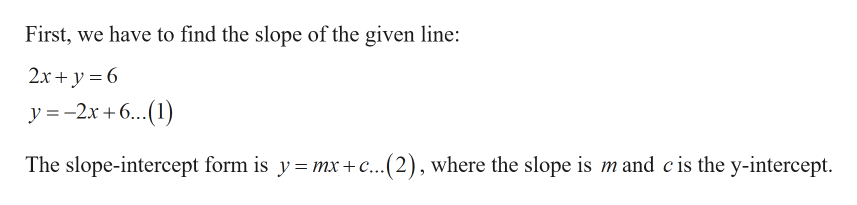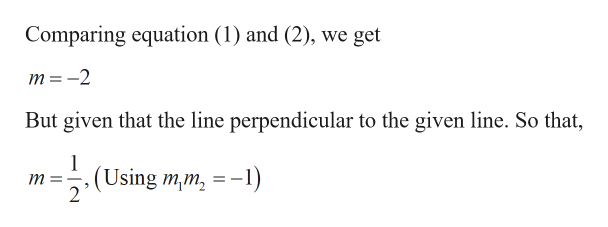# Determine the equation of the line that contains the point (2, -3) and is perpendicular to the line 2 x + y = 6.

Question
2 views

Determine the equation of the line that contains the point (2, -3) and is perpendicular to the line 2 x + y = 6.

check_circle

Step 1

Given:

Step 2

Calculation:

To find the equation of the line:help_outlineImage TranscriptioncloseFirst, we have to find the slope of the given line: 2x + y = 6 y =-2x +6...(1) The slope-intercept form is y= mx + c...(2), where the slope is m and cis the y-intercept. fullscreen
Step 3help_outlineImage TranscriptioncloseComparing equation (1) and (2), we get :-2 But given that the line perpendicular to the given line. So that, 1 (Using m,m, = -1) %3D fullscreen

### Want to see the full answer?

See Solution

#### Want to see this answer and more?

Solutions are written by subject experts who are available 24/7. Questions are typically answered within 1 hour.*

See Solution
*Response times may vary by subject and question.
Tagged in

### Other